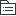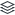# 图案设计

|
17

## 相关模板推荐

•## 图案设计 —— 作品大纲

•新石器时代
• 仰韶文化（彩陶艺术）
• 对称
• 条理
• 反复
• 韵律
• 节奏
• 均衡
• 表现形式
• 二方连续
• 四方连续
•商周时期
• 青铜器
• 风格
• 凝重，严肃，神秘
• 意象
• 部落动物
• 图腾符号
• “less is more”空间留白，神秘
•春秋战国时期
• 总体风格：清新，灵活，轻快，抽象，自由
• 漆器
• 纹样
• 纤细（形象生动）弧线形，斜线形
• 动物纹，云气纹，几何纹
• 题材
• 狩猎
• 宴饮
• 农作
• 战争
• 风格
• 封闭到开放
• 造型
• 变形到写实
• 轮廓
• 直线主调到自由曲线主调
• 艺术格调
• 静止到活泼生动
• 玉器（春秋）
• 玦，璜
•汉代
• 总体风格：简洁，整体
• 画像石，画像砖
• 题材
• 封建贵族生活
• 车马
• 河伯出行
• 人物
• 主题
• 忠孝节义
• 金属工艺
• 铜镜，铜器，浮雕
• 金银猎兽
• 鎏金，金银器
• 颜色：黑红
•南北朝
• 题材
• 石狮，祥禽，瑞兽
• 植物
• 莲花，忍冬草
• 人物
• 飞天，乘龙骑虎的神仙，名流高士出行讲道说教，舞乐伎，武士
• 产品
• 北齐佛像
• 端庄秀丽
• 印纹砖
• 模具压印
• 阴刻
• 模具
• 阳刻
• 砖坯
•唐朝
• 总体风格：精致丰满华贵
• 主题装饰：鸟兽植物花卉
• 典型图案：宝相花
• 朵花花头为标本
• 镶嵌于花瓣轮廓线及花心中心，花心轮廓线位置
• 风格
• 饱满，富有内在张力，表现手法统一
•宋朝
• 总体风格：淡雅，清秀，凝重
• 官窑
• 御窑瓷
• 官窑瓷
• 民窑
• 定窑，汝窑，钧窑，磁州窑
•元朝
• 总体风格：灵活自由，粗放（体相，变形）
• 青花瓷
• 釉里红
• 题材
• 龙饰
•明清时期
• 总体风格：中西结合过于纤细，繁缛（市场制约）
• 景泰蓝（明）
• 青花瓷
• 斗彩（明）
• 五彩（明清）
• 粉彩（清）
• 珐琅（清）
• 东阳木雕
• 苏绣，湘绣
•西方艺术风格
• 巴洛克（意大利）（男性化）
• 动感不规则
• 不重实用，豪华华丽
• 彰显宗教贵族的尊严
• 色彩鲜艳
• 放弃古典形式，具有综合性，强调艺术形式的统一手段
• 空间深度大，立体感强
• 远离生活与时代（天顶画）人形象微不足道
• 运动与变化
• 著名人物：弗拉戈纳尔
• 《圣德丽莎的幻觉》
• 洛可可（女性化）
• “曲线”趣味
• C形，S形，漩涡曲线
• 非对称
• 轻快优雅
• 爱的主题
• 色彩柔和
• 相同点
• 17C—18C
• 经历文艺复兴
• 贵族艺术
• 为宗教服务
• 受中国传统的影响，矫饰主义
•欧洲新艺术时期
• 时期
• 19C末20C初
• 性质
• 装饰艺术与运动
• 应用
• 家具，产品装饰，插画，建筑雕塑
• 背景
• 工艺美术运动
• 普法战争后，欧洲社会安定，经济平稳，新艺术形式产生的迫切性
• 风格
• 扎实与前卫
• 纹样特点
• 汲取大自然的装饰艺术，与自然融为一体，花草纹
• 完全放弃传统装饰风格，开创全新自然装饰风格
• 不存在直线平面，突出有机的曲线风格
• 装饰受到东方风格的影响
• 著名人物
• 威廉莫里斯
• 提出装饰与结构因素的协调
•日本浮世绘
• 时期
• 江户时代【同清代】
• 风格
• 宫廷
• 美艳，鲜艳
• 町人文化
• 市民文化